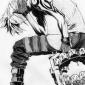# plane figures

2 posts / 0 new
Ei Zeeplane figures

A rectangle ABCD, which measures 9 ft is folded once perpendicular to diagonal AC such that the opposite vertices A and C coincide. Find the length of the fold.

Jhun VertWhat is the dimension of the other side of rectangle?

• Mathematics inside the configured delimiters is rendered by MathJax. The default math delimiters are $$...$$ and $...$ for displayed mathematics, and $...$ and $...$ for in-line mathematics.